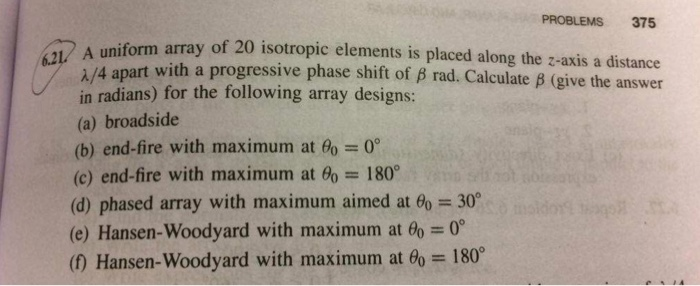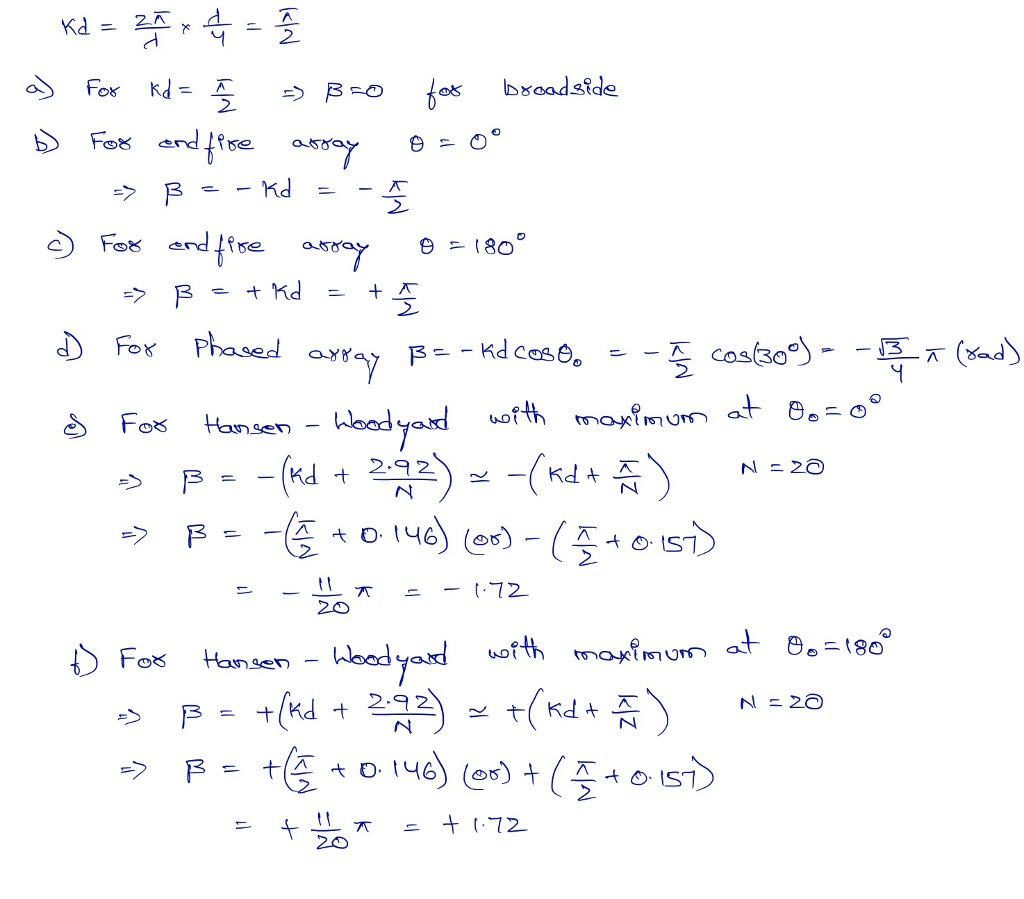# Question & Answer: Antenna theory Analysis and Design 3rd edition chapter 6 number 21…..Antenna theory Analysis and Design 3rd edition chapter 6 number 21

A uniform array of 20 isotropic elements is placed along the z-axis a distance lambda/4 apart with a progressive phase shift of beta rad. Calculate beta (give the answer in radians) for the following array designs: (a) broadside (b) end-fire with maximum at theta_0 = 0 degree (c) end-fire with maximum at theta_0 = 180 degree (d) phased array with maximum aimed at theta_0 = 30 degree (e) Hansen-Woodyard with maximum at theta_0 = 0 degree (f) Hansen-Woodyard with maximum at theta_0 = 180 degree